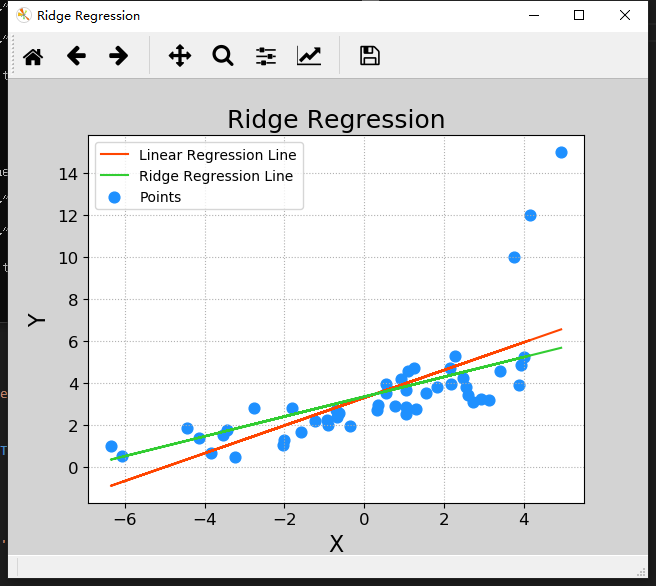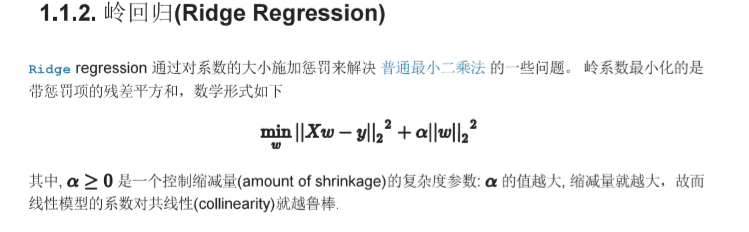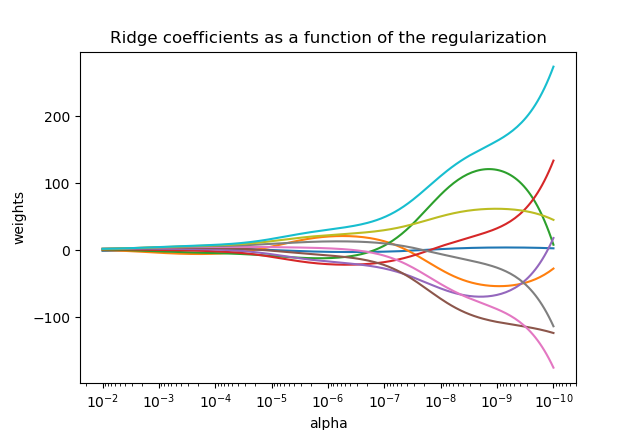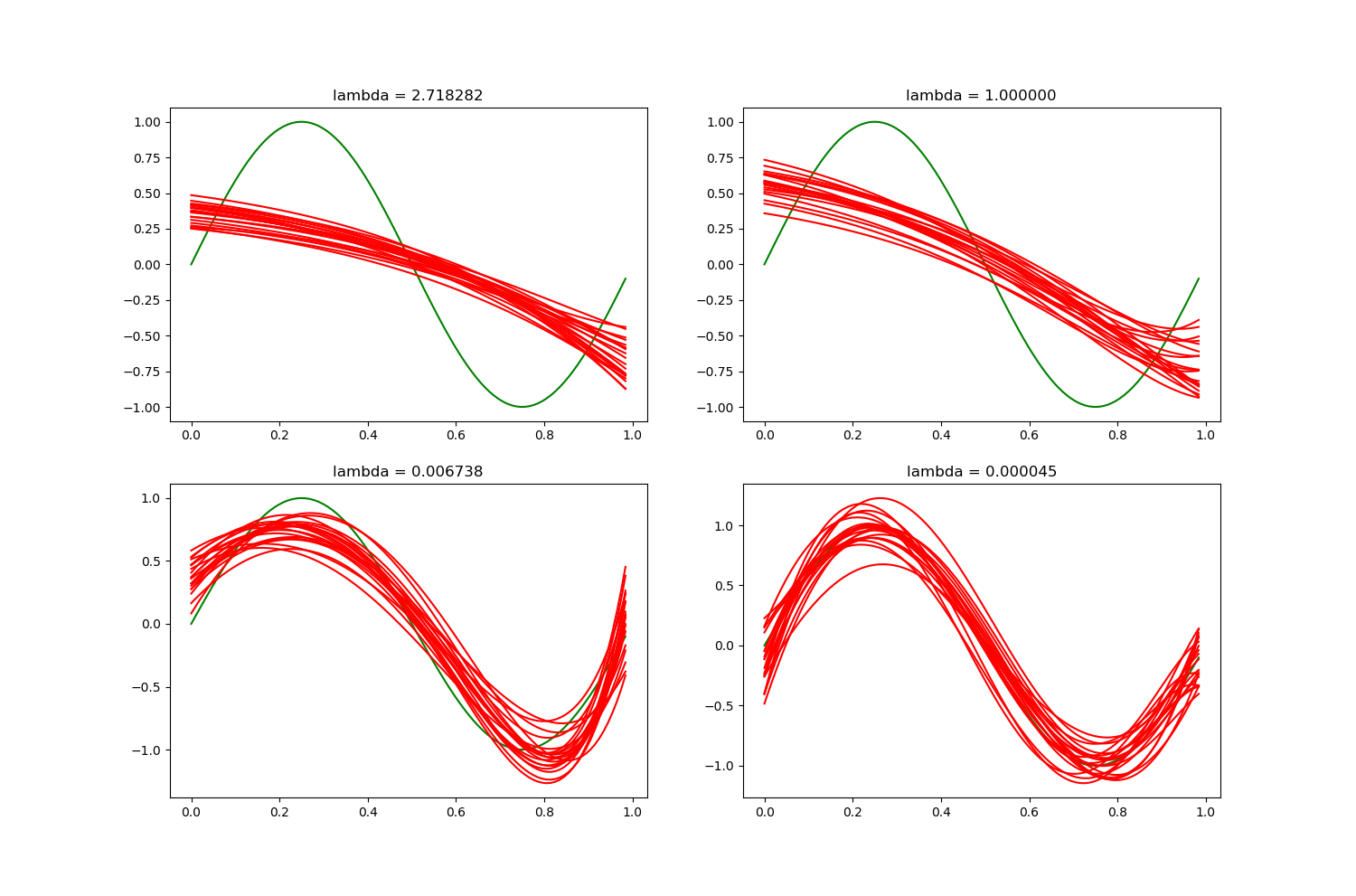• 2021-06-17 17:46:07

## 本文所用文件的链接

链接：https://pan.baidu.com/s/1RWNVHuXMQleOrEi5vig_bQ
提取码：p57s

### 岭回归

普通的线性回归模型使用基于梯度下降的最小二乘法, 在最小化损失函数的前提下, 寻找最优模型参数. 在此过程中, 包括少数的异常样本在内的全部训练数据都会对最终的模型参数造成相等程度的影响, 并且异常值对模型所带来的影响无法在训练过程中被识别出来. 为此, 岭回归在模型迭代过程中增加了正则项, 用来限制模型参数对异常样本的匹配程度, 进而提高模型面对大多数正常样本的拟合精度.

import sklearn.linear_model as lm
model = lm.Ridge(
正则强度,
fit_intercept=是否训练截距,
max_iter=最大迭代次数
)
model.fit(输入,输出)
预测输出 = model.predict(输入)


该案例利用线性回归和领回归分别训练了同一组数据，很明显，岭回归能够不受异常数据的影响，训练的直线更符合大多数数据的变换规律。而线性回归则明显受到了异常数据的影响，这个问题在机器学习中称为过拟合，后续的复杂模型中会有各种方式来避免过拟合，而岭回归就是防止线性回归过拟合的一种方式。

"""
岭回归
"""
import numpy as np
import sklearn.linear_model as lm
import matplotlib.pyplot as mp
import sklearn.metrics as sm

delimiter=',', usecols=(0,1),
unpack=True)
# 把x改为n行1列  这样才可以作为输入交给模型训练
x = x.reshape(-1, 1)
mp.figure('Ridge Regression', facecolor='lightgray')
mp.title('Ridge Regression', fontsize=18)
mp.xlabel('X', fontsize=16)
mp.ylabel('Y', fontsize=16)
mp.tick_params(labelsize=12)
mp.grid(linestyle=':')
mp.scatter(x, y, s=60, c='dodgerblue',
label='Points')

# 使用线性回归, 绘制回归线
model = lm.LinearRegression()
model.fit(x, y)
pred_y = model.predict(x)
mp.plot(x, pred_y, c='orangered',
label='Linear Regression Line')

# 使用岭回归, 绘制回归线
model = lm.Ridge(150, fit_intercept=True,
max_iter=1000)
model.fit(x, y)
pred_y = model.predict(x)
mp.plot(x, pred_y, c='limegreen',
label='Ridge Regression Line')

mp.legend()
mp.show()机器学习 深度学习 人工智能
更多相关内容
• 代码功能介绍在《初探 岭回归 LASSO回归 （python 实现）》中有详细的介绍，文章中若有不正确的，也希望能够不吝赐教，相互学习。
• ## python实现岭回归

千次阅读 2019-05-07 22:52:37
岭回归实现（最小二乘法的带惩罚项版） # 代码实现 import numpy as np import matplotlib.pyplot as plt from sklearn import linear_model def main(): # X 是一个 10x10 的 希尔伯特矩阵(Hilbert matrix) X = ...

# 岭回归实现（最小二乘法的带惩罚项版）# 代码实现

import numpy as np
import matplotlib.pyplot as plt
from sklearn import linear_model

def main():
# X 是一个 10x10 的 希尔伯特矩阵(Hilbert matrix)
X = 1. / (np.arange(1, 11) + np.arange(0, 10)[:, np.newaxis])
#十个1的向量
y = np.ones(10)

# 计算路径(Compute paths)
n_alphas = 200
alphas = np.logspace(-10, -2, n_alphas)

coefs = []
for a in alphas:
ridge = linear_model.Ridge(alpha=a, fit_intercept=False)    #每个循环都要重新实例化一个estimator对象
ridge.fit(X, y)
coefs.append(ridge.coef_)
# 展示结果
ax = plt.gca()
ax.plot(alphas, coefs)
ax.set_xscale('log')
ax.set_xlim(ax.get_xlim()[::-1])  # 反转数轴，越靠左边 alpha 越大，正则化也越厉害
plt.xlabel('alpha')
plt.ylabel('weights')
plt.title('Ridge coefficients as a function of the regularization')
plt.axis('tight')
plt.show()
if __name__='__main__':
main()


# 运行结果展开全文• 基于python实现岭回归算法RidgeRegression
• 在介绍岭回归算法与Lasso回归算法之前，先要回顾一下线性回归算法。根据线性回归模型的参数估计公式可知可知，得到的前提是矩阵可逆。换句话说就是样本各个特征(自变量)之间线性无关。然而在实际问题中，常常会出现...

在介绍岭回归算法与Lasso回归算法之前，先要回顾一下线性回归算法。根据线性回归模型的参数估计公式可知可知，得到的前提是矩阵可逆。换句话说就是样本各个特征(自变量)之间线性无关。然而在实际问题中，常常会出现特征之间出现多重共线性的情况，使得行列式的值接近于0，最终造成回归系数无解或者无意义。

为了解决这个问题，岭回归算法的方法是在线性回归模型的目标函数之上添加一个l2的正则项，进而使得模型的回归系数有解。具体的岭回归目标函数可表示为如下：在Python中，岭回归算法的实现方法如下。

在Python中sklearn算法包已经实现了所有基本机器学习的算法。直接

from sklearn.linear_model import Ridge,RidgeCV

就可以调用该算法。

Iris数据集的加载与预处理已在前文中介绍过，此处不再累述。定义岭回归算法的方法为如下：

rigdeCV = RidgeCV(alphas=Lambdas,normalize=True,scoring='neg_mean_squared_error',cv=15)

其中alphas用于指定多个λ值的元组或数组对象，默认该参数包含0.1、1和10三个值。normalize指是否对数据集进行归一化处理。scoring指的是用于评估模型的度量方法，此处为RMSE均分误差。cv指交叉验证的重数。

此处我使用的是15重交叉验证法确定的λ值。上面Lambdas为logspace方法生成的数组。

Lambdas = np.logspace(-5,2,200)

最后训练，测试：

rigdeCV.fit(X_train,Y_train)

predictedResult= rigdeCV.predict(X_test)

注意此处predictedResult为Series不是List。

此时岭回归算法分类正确率为0.8。岭回归算法固然解决了线性回归模型中矩阵不可逆的问题。但岭回归算法无论如何会保留建模时所有的变量，无法降低模型的复杂度。为了克服岭回归算法的缺点，Lasso回归算法被提了出来。

与岭回归算法类似，Lasso回归算法同样属于缩减性估计。而且在回归系数的缩减过程中，可以将一些不重要的回归系数缩减为0，以打到变量筛选的目的。Lasso回归算法的目标函数为如下：具体在Python上实现的方式与岭回归算法类似，先导入liner_model中的LassoCV类，然后就可以使用：

lasso_cv = LassoCV(alphas=Lambdas,normalize=True,cv=10,max_iter=10000)

这里max_iter指的是模型最大迭代次数。其它参数与岭回归类似。

此时Lasso回归算法的准确率为0.8。由于岭回归算法和Lasso回归算法的相似性，在本次实验中，二者的分类结果完全相同，分类准确率同为0.8。考虑到线性回归并不是特别适合分类问题，所以这种结果也可以接受。下一步的学习方向是GBDT以及多种算法的综合模型。

展开全文• ## Python实现岭回归

千次阅读 2018-09-25 13:23:07
题目： ...在每组数据集上用具有不同的7阶多项式进行岭回归拟合 import numpy as np import matplotlib.pyplot as plt from tkinter import _flatten x_arange = 0.041 * np.arange(0, 25, 1) #每...

## 题目：

随机产生100组数据集，每组数据集包含25个点，每个点满足：，这里，e是添加的高斯噪声。在每组数据集上用具有不同的7阶多项式进行岭回归拟合

import numpy as np
import matplotlib.pyplot as plt
from tkinter import _flatten

x_arange = 0.041 * np.arange(0, 25, 1) #每组数据的25个点
y_True = np.sin(2 * np.pi * x_arange)  #每个数据点对应的值（没有添加噪声）
y_Noise = np.zeros(y_True.shape)       #添加噪声的值
x_Prec = np.linspace(0, 24*0.041, 100) #画图范围

mu = 0  #噪声的mu值
sigma = 0.3  #噪声的sigma值
Num = 100  #100组数据集
n = 8  #7阶多项式
lamda = [np.exp(1), np.exp(0), np.exp(-5), np.exp(-10)]  #不同的lambda值
phi = np.mat(np.zeros((x_arange.size, n)))  #phi矩阵
x = np.mat(x_arange).T  #输入数据矩阵

#phi矩阵运算
for i_n in range(n):
for y_n in range(x_arange.size):
phi[y_n, i_n] = x[y_n, 0] ** i_n

plt.figure(figsize=(15, 10))
index = 221
for i_lamda in lamda:
plt.subplot(index)
index += 1
plt.title("lambda = %f" % i_lamda)
plt.plot(x_Prec, np.sin(2 * np.pi * x_Prec), color='g')
for k in range(Num):
for i in range(x_arange.size):
y_Noise[i] = y_True[i] + np.random.normal(mu, sigma)
y = np.mat(y_Noise).T
#求解w参数
W = (phi.T * phi + i_lamda*np.eye(n)).I * phi.T * y

ploy = list(_flatten(W.T.tolist()))
ploy.reverse()
p = np.poly1d(ploy)
if k%5==0:  #只画20条曲线
plt.plot(x_Prec, p(x_Prec), color='r')
plt.show()


结果：展开全文• 机器学习中线性回归以及岭回归算法的实现Python版本
• 4 Python实现 4.1 代码 4.2 结果 5 正则化 1 概述 1.1 线性回归 对于一般地线性回归问题，参数的求解采用的是最小二乘法，其目标函数如下： 参数w的求解，也可以使用如下矩阵方法进行： ...人工智能
• 常见的回归：线性回归、岭回归、非线性回归 回归拟合目标：计算自变量与因变量关系的函数参数 通过不断拟合缩小预测值与真实值的差距：最终使得这个差距（误差项）成为一组均值为0，方差为1的随机数。 2、损失函数 ...
• 岭回归的原理：首先要了解最小二乘法的回归原理设有多重线性回归模型 y=Xβ+ε ,参数β的最小二乘估计为当自变量间存在多重共线性,|X'X|≈0时，设想|X'X|给加上一个正常数矩阵(k>0)那么|X'X|+kI 接近奇异的程度就...
• 第一部分 转换数据，把从一个文件夹下的所有文件写到一个大文件 ...#该模块，实现转换数据的功能 #全局变量 DATA=pd.DataFrame() def readfile(filepath): externfiles=os.listdir(filepath) externfiles.sort()...
• ## python-岭回归模型

千次阅读 2020-12-23 20:49:36
岭回归模型的应用 ①可视化方法确定值 当回归系数随着值的增加而趋近于稳定的点时就是所要寻找的值 import pandas as pd import numpy as np import matplotlib.pyplot as plt import matplotlib from sklearn ...机器学习
• [机器学习-5]岭回归[L2正则化]及python实现（Ridge Regression）【L2】前言题目岭回归（Ridge Regression）k-fold validation(1)实现（realization）(2)实现 前言 ！！实现直接跳转实现 这章本来是为了接前面的过...
• 岭回归与多项式回归的最大区别就是损失函数上的区别。岭回归的代价函数如下：为了方便计算导数，通常也会写成以下形式：上述式子中w为长度为n的向量，不包括偏置项的系数 θ0，θ是长度为n+1的向量，包括偏置项系数...
• 接着上节讨论，岭回归也是一种用于回归的线性模型，因此它的预测公式与普通的最小二乘法相同。但在岭回归中，对系数(w)的选择不仅要在训练数据上得到好的预测结果，...下面我们来看岭回归在linear_model.Ridge中实现...
• - iPytLab前言继续线性回归的总结, 本文主要介绍两种线性回归的缩减(shrinkage)方法的基础知识: 岭回归(Ridge Regression)和LASSO(Least Absolute Shrinkage and Selection Operator)并对其进行了Python实现。...
• 一、标准线性回归   在线性回归中我们要求的参数为： 详细的推导可以参见：...所以代码实现主要就是实现上式，python代码如下： import numpy as np import pandas as pd import matplotlib.pyplo
• 用于岭回归python代码（已实现以预测下个月的CO2浓度） 资料可用性 文件 Ridge.py ：标准函数和Ridge回归函数window_make.py ：使用滑动窗口方法制作大小为p（窗口大小）的时间序列列表。 Final_version.ipynb ：...
• 线性回归 算法简介 线性回归，就是利用数理统计中的回归分析，来确定两种或两种以上变量间，相互依赖的定量关系的一种统计分析方法。 线性回归试图学得： 求解w和b的关键在于衡量f(xi)与yi之间的差别.由于均方...机器学习
• x_{im}) \, {函数值 y_i}\]每个样本有m个变量回归面$f(x_i) = x_i^T \omega +b$$$\omega = (\omega_1; \omega_2; ...; \omega_m)$$$\hat x_i=(x_{i1};x_{i2}; ...; x_{im}; 1)$\[\hat \omega = (...
• 岭回归介绍及实现1 岭回归的引入2 岭回归的原理2.1 原理介绍2.2 原理代码实现3 API 实现 1 岭回归的引入 在线性回归-正规方程和梯度下降中，我们介绍了基于正规方程或者梯度下降的优化算法，来寻找最优解。 在正规...机器学习
• 作为一名曾经研究过人工神经网络的菜鸟，前两天刚听说有岭回归估计和LASSO估计，统计学老师也布置了作业，然，在不甚理解的情况下，用Python写了一下，也不知是否正确。不合适的地方请不吝赐教。 作业如下： x，...岭估计
• 基于Python岭回归与LASSO回归模型介绍及实践 这是一篇学习的总结笔记 参考自《从零开始学数据分析与挖掘》 [中]刘顺祥 著 完整代码及实践所用数据集等资料放置于：Github 岭回归与LASSO回归模型是线性回归...机器学习 数据挖掘
• 概率统计笔记：贝叶斯线性回归_UQI-LIUWJ的博客-CSDN博客 1 数据集部分 1.1 创建数据集 import matplotlib.pyplot as plt a_true = 2 b_true = 1 tau_true = 1 n = 50 x = np.random.uniform(low = 0, high = ...机器学习
• 在介绍Ridge和Lasso回归之前，我们先了解一下正则化 过拟合和欠拟合 (1) under fit：还没有拟合到位，训练集和测试集的准确率都还没有到达最高。学的还不 到位。 (2) over fit：拟合过度，训练集的准确率升高的...机器学习
• 标准方程法的改进–岭回归算法的python实现实现 前言： 岭回归相比标准方程法就是多了一个岭系数，目的是防止数据不可逆导致的参数无法估计的问题，关于岭回归的具体理论请点击此处，到我的一篇博文中查看 一、...机器学习 线性回归模型
• 岭回归，又叫吉洪诺夫正则化，是由Hoerl和Kennard于1970年提出的是一种专用于共线性数据分析的有偏估计回归法。岭回归实际上是一种改良的最小二乘估计法，具有L2正则化的线性最小二乘法。回归算法的，本质就是为了...机器学习 人工智能 算法...